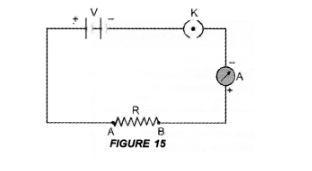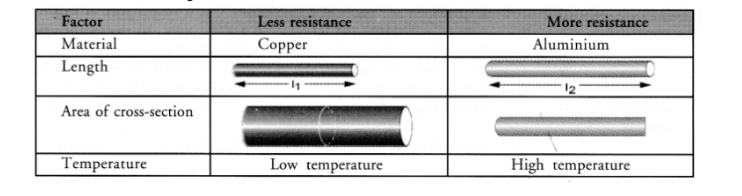# What is electrical resistivity of a material ?

Question:

What is electrical resistivity of a material ? What is its unit ?

Describe an experiment to study the factors on which the resistance of conducting wire depends. (CBSE 2012)

Solution:

For Electrical resistivity: Electrical resistivity of a material is defined as the resistance of an object (made of the material) of unit length and unit

area of cross-section.

Unit of electrical resistivity is ohm-metre.

For experiment:

Connect the various electrical components as shown in figure 15.1. Dependence of length of a conductor:
Take a copper wire of length l and connect it between the terminals A and B. Note the reading of ammeter. Now take another copper wire of same area of cross-section but of length 2l. Connect it between the terminals A and B by disconnecting the previous wire. Again, note the reading of ammeter. It will be found that the reading of ammeter (i.e., electric current) in the second case is half of the reading of ammeter in the first case. Since R = V/I, so resistance of second wire is double than the resistance of the first wire. Thus, resistance of a conductor is directly proportional to the length of the conductor,
i.e      resistance ∝ length of the conductor
Thus, more is the length of a conductor, more is its resistance.
Thus, the resistance of’a conductor is ‘inversely proportional to the area of cross -section of the conductor.
2. Dependence on area of cross-section of a conductor:
Now take two copper wires of same length but of different area of cross-sections. Let area of cross-section of first wire is more than the area of cross-section of the second wire. Connect first wire between the terminals A and B in the circuit shown in figure 15. Note the reading of ammeter. Now disconnect the first wire and connect the second wire between the terminals A and B. Again note the reading of the ammeter. It will be found that the reading of ammeter (i.e. electric current) is more when first wire (i.e. thick wire) is connected between A and B than the reading of the ammeter when second wire (i.e. thin wire) is connected between the terminals A and B.

i.e. resistance $\propto \frac{1}{\text { area of cross - section of the conductor }}$

or $\mathrm{R} \propto \frac{1}{\mathrm{~A}}$

Thus, the resistance of a thin wire is more than the resistance of a thick wire.

3. Effect of the Nature of material:

Take two identical wires, one of copper and other of aluminium. Connect the copper wire between the terminals A and B. Note the reading of ammeter. Now, connect the aluminium wire between the terminals $A$ and $B$. Again note the reading of ammeter. It is found that the reading of ammeter when copper wire is connected in the circuit is more than the reading of the ammeter when aluminium wire is connected in the circuit.

Therefore, resistance of copper wire is less than the resistance of aluminium wire. Hence, resistance of a wire or a conductor depends upon the nature of the material of the conductor.

4. Effect of temperature of conductor:

If the temperature of a metallic conductor connected in the circuit increases, its resistance increases. Thus, factors on which resistance of a conductor depends are :

1. its length,
2. its area of cross-section,
3. the nature of its material and
4. its temperature.

The various factors affecting resistance of a conductor are given in table 1.
Table 1. Factors affecting resistance of a conductor: